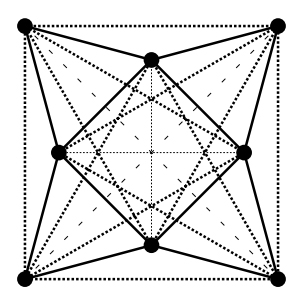# Дни комбинаторики и геометрии I

в МФТИ
26 - 27 Ноября, 2019
Долгопрудный
МФТИ
The conference aims to be a meeting point for combinatorialists and geometers, where they will be able to present and discuss their current research, as well as give broader scope lectures accessible to interested PhD and master students.
November 26
Roman Karasev
Envy-free divisions and mapping degrees
joint work with Sergey Avvakumov, Arkadiy Skopenkov, and Sergey Kudrya

We discuss some classical problems of mathematical economics, in particular, so-called envy-free division problems. The classical approach to some of such problems reduces to considering continuous maps of a simplex to itself and finding sufficient conditions when this map hits the center of the simplex. The mere continuity is not sufficient for such a conclusion, the usual assumption (for example, in the Knaster–Kuratowski–Mazurkiewicz theorem and the Gale theorem) is a boundary condition.

We try to replace the boundary condition by a certain equivariance condition under all permutations, or a weaker condition of "pseudo-equivariance'', which has a certain real-life meaning for the problem of partitioning a segment and distributing the parts among the players. It turns out that we can guarantee the existence of a solution for the segment partition problem when the number of players is a prime power; and we may produce instances of the problem without a solution otherwise. The case of three players was solved previously by Segal–Halevi, the prime case and the case of four players were solved by Meunier and Zerbib.

Going back to the true equivariance setting, we provide, in the case when the number of players is not a prime power and not twice a prime power, the counterexamples showing that the topological configuration space / test map scheme for a wide class of equipartition problems fails and some envy-free division problems have a counterexample. Moreover, this is also applicable to building stronger counterexamples for the topological Tverberg conjecture.

Nikita Kalinin
The number π and summation by SL(2, $Z$)
joint work with Mikhail Shkolnikov

The sum (resp. the sum of the squares) of the defects in the triangle inequalities for the area one lattice parallelograms in the first quadrant has a surprisingly simple expression. Namely, let $$f(a, b, c, d) = \sqrt{a^2 + b^2} + \sqrt{c^2 + d^2} - \sqrt{(a+c)^2 + (b+d)^2}.$$ Then, $$\sum f(a, b, c, d) = 2,$$ $$\sum f(a, b, c, d)^2 = 2 - \frac{\pi}{2},$$ where the sum runs by all $a, b, c, d \in Z \geq 0$ such that $ad − bc = 1$. This paper is devoted to the proof of these formulae. We also discuss possible directions in study of this phenomena.## Location

The conference will take place in MIPT, Dolgoprudny.
Trains from Moscow
To get to the MIPT campus from Moscow you can catch a suburban train at Savyolovskaya, Timiryazevskaya or Okruzhnaya platforms. Platforms are close to the metro stations with the same names. The ride on the train takes from 15 to 30 minutes.
Buses from Moscow
There are buses to Dolgoprudny from Altufyevo, Skhodnenskaya or Khovrino metro stations. The ride on the bus takes from 30 to 50 minutes.
Taxi from Moscow
You can also take a taxi. Easiest way to do so is to use Yandex Taxi service on web or by phone +7 (495) 999 99 99.